## 数学推导 傅里叶级数与傅里叶变换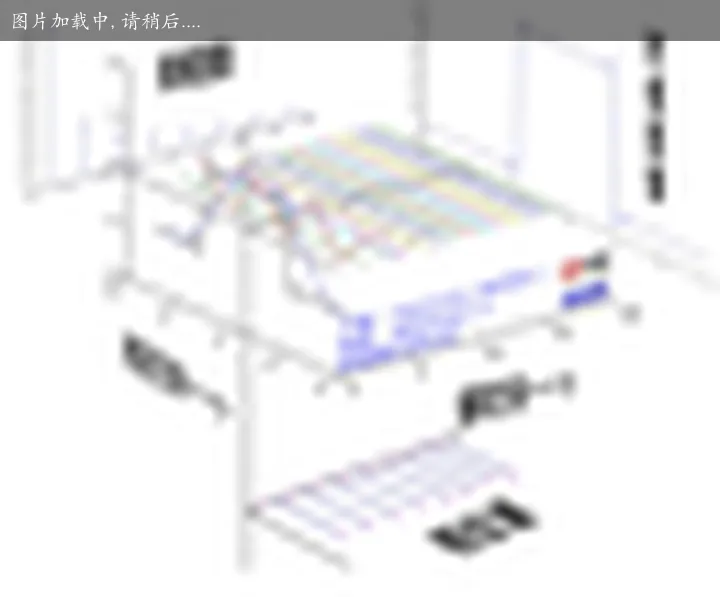Part1. 三角函数的正交性 https://www.bilibili.com/video/BV1Et411R78v

Part2. 周期为 $2\varPi$ 的函数展开 https://www.bilibili.com/video/BV17t411d7hm

Part3. 周期为 $2L$ 的函数展开 https://www.bilibili.com/video/BV1wb411K7Kp

Part4. 傅里叶级数的复数形式 https://www.bilibili.com/video/BV13b411P7mU

Part5. 从傅里叶级数推导傅里叶变换 https://www.bilibili.com/video/BV1jt411U7Bp

Part6. 总结与闲话 https://www.bilibili.com/video/BV1St41117fH

“珂学原理”No. 26“拉普拉斯变换了什么？”### 复数的物理意义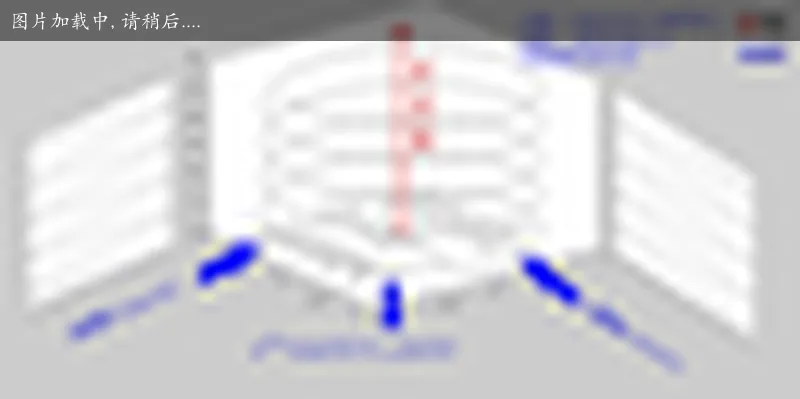### 傅里叶变换（Fourier Transformation）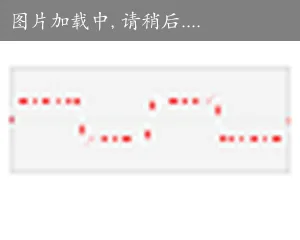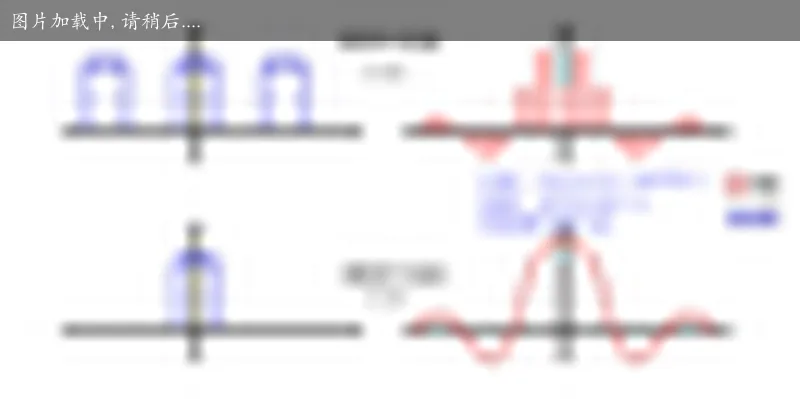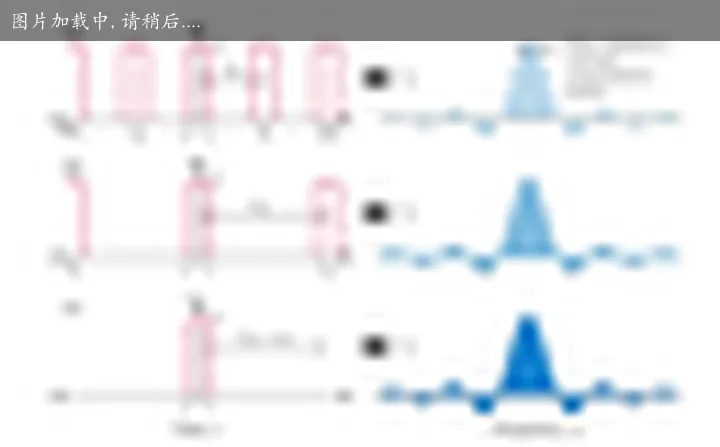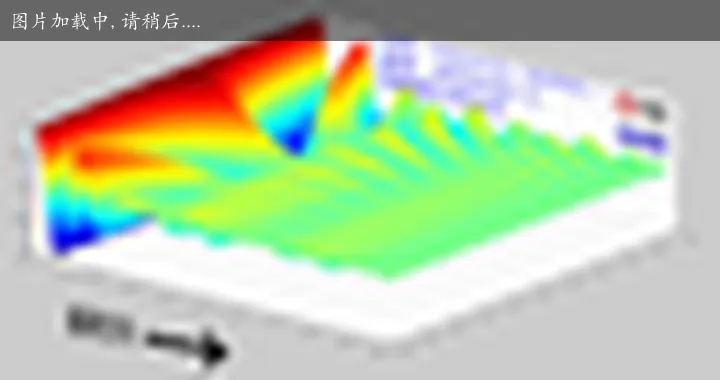3D 动画详细解释傅里叶与拉普拉斯变换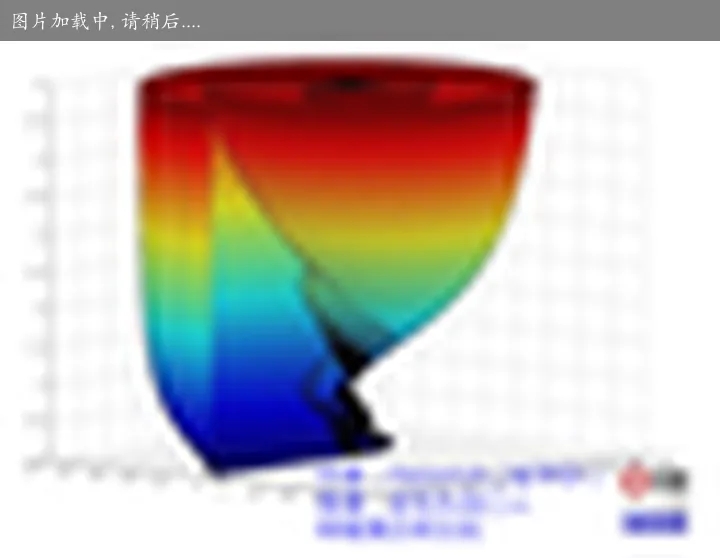## 图像傅里叶变换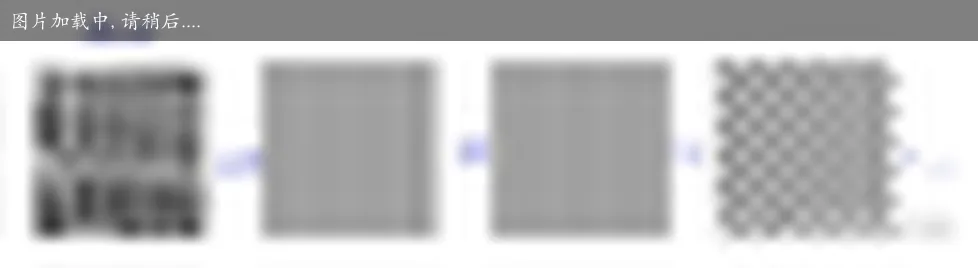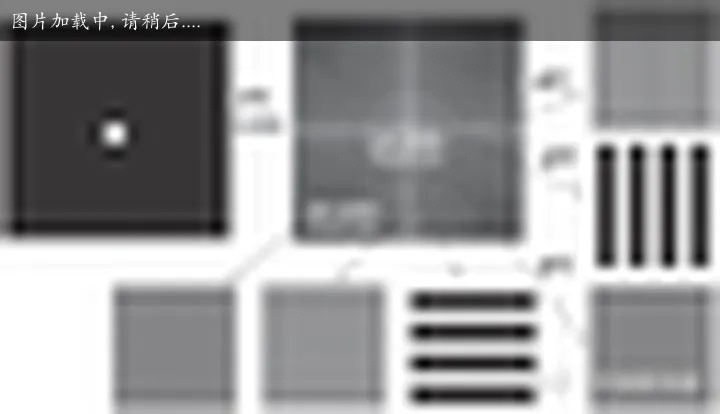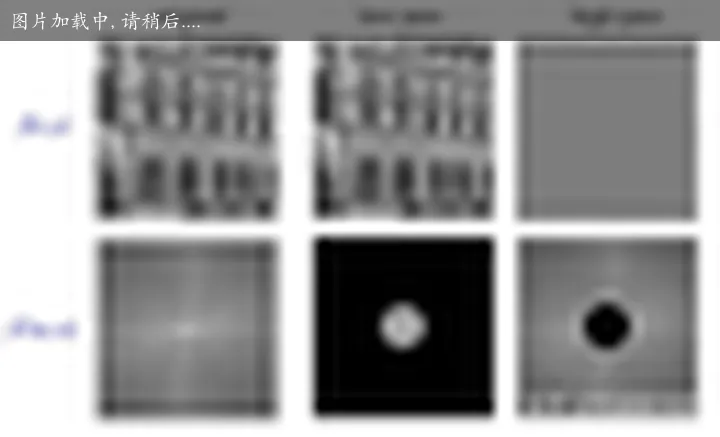k 空间中的 phase 保留的是位置信息，如果没有幅度或者，幅度错误，只是对比度会不同；而 mag 保留的是强度信息，没有 phase 的话，强度相加也是按照周期性相加。

## 傅里叶变换、拉普拉斯变换、Z 变换

• 傅里叶变换是把信号从【时域】变换到【频域】。
• 拉普拉斯变换是想办法把不满足傅里叶变换条件的【连续信号】从时域变换到频域。
• Z 变换是想办法把不满足傅里叶变换条件的【离散信号】从时域变换到频域。

## 应用举例

### 螺旋 CT 成像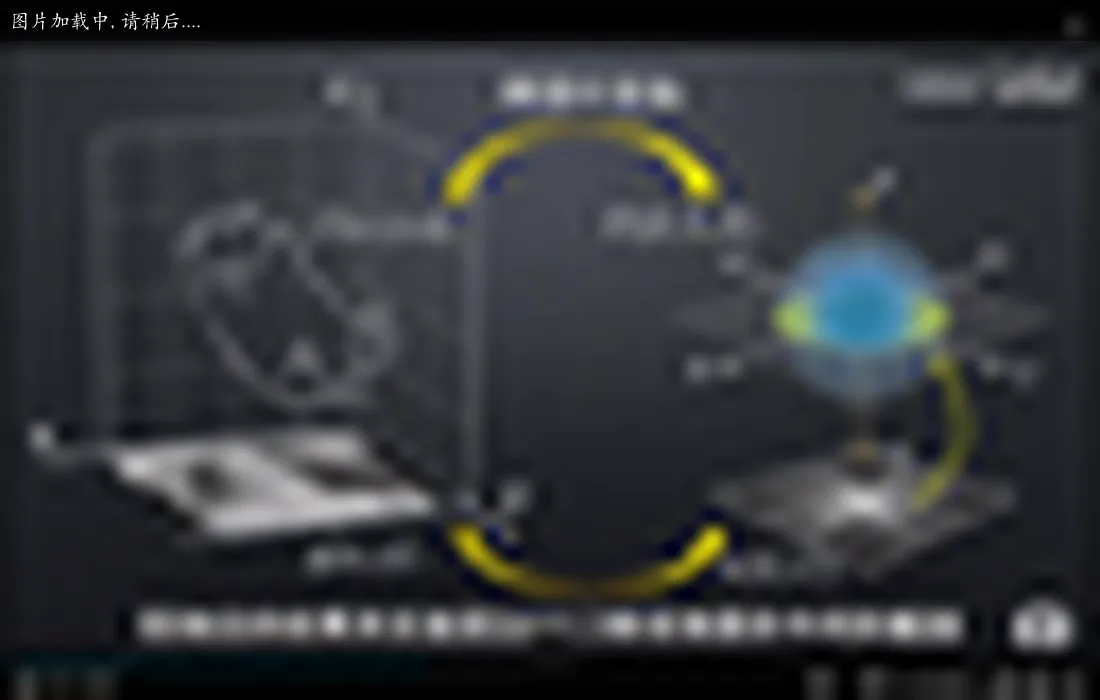### 指纹去噪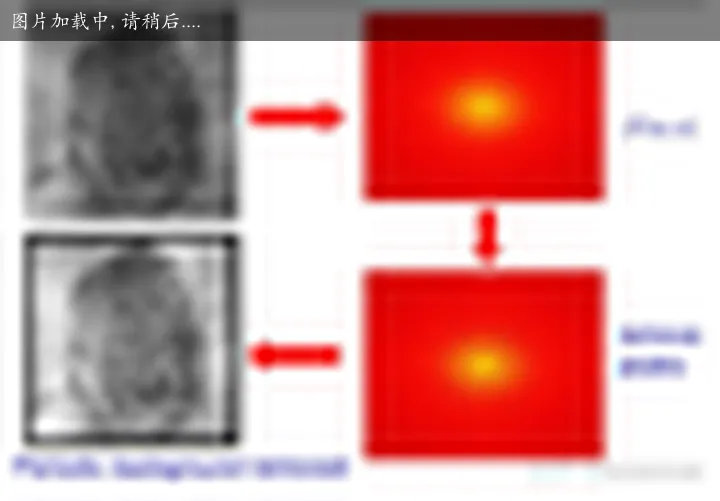### 天体表面去噪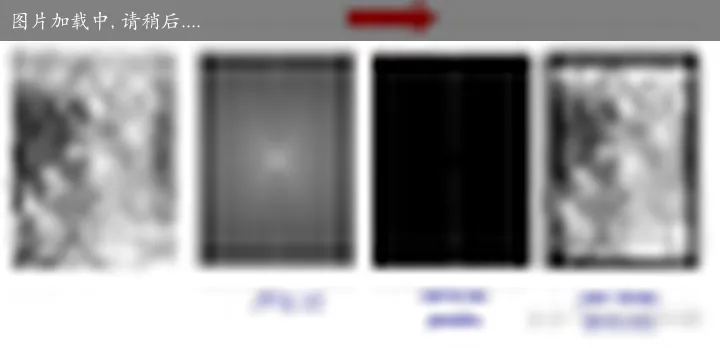## 代码实验

#encoding=utf8
import numpy as np
import matplotlib.pyplot as plt
from scipy import fftpack
from scipy import ndimage
import cv2

def showgray(im, title):
plt.figure("fft_"+title)
plt.imshow(im, plt.cm.gray)
plt.title(title)
plt.show()

# Show the results
def plotSpectrum(imfft, title):
plt.figure("fft_"+title)
from matplotlib.colors import LogNorm
# A logarithmic colormap
#plt.imshow(np.abs(imfft), norm=LogNorm(vmin=5))
magnitudeSpectrum = 20*np.log(np.abs(imfft))
plt.imshow(magnitudeSpectrum)
plt.colorbar()
plt.title(title)
plt.show()

def plotSpectrum2(srcimg, fshift, title):
plt.figure("fft_"+title)
magnitudeSpectrum = 20*np.log(np.abs(fshift))
plt.subplot(121), plt.imshow(srcimg, cmap = 'gray')
plt.title('Input Image'), plt.xticks([]), plt.yticks([])
plt.subplot(122), plt.imshow(magnitudeSpectrum, cmap = 'gray')
plt.title('Magnitude Spectrum'), plt.xticks([]), plt.yticks([])
plt.show()

if __name__ == "__main__":
#showgray(im, 'Original image')

imfft = fftpack.fft2(im)
#plotSpectrum(imfft, 'Fourier transform')
plotSpectrum2(im, imfft, "Original image")

keepFraction = 0.1
imfft2 = imfft.copy()
r, c = imfft2.shape
imfft2[int(r*keepFraction):int(r*(1-keepFraction)), ...] = 0
imfft2[..., int(c*keepFraction):int(c*(1-keepFraction))] = 0
#plotSpectrum(imfft2, 'Filtered Spectrum')

imnew = fftpack.ifft2(imfft2).real
#showgray(imnew, 'Reconstructed Image')
plotSpectrum2(imnew, imfft2, "Reconstructed Image")

imblur = ndimage.gaussian_filter(im, 4)
showgray(imblur, 'Blurred image')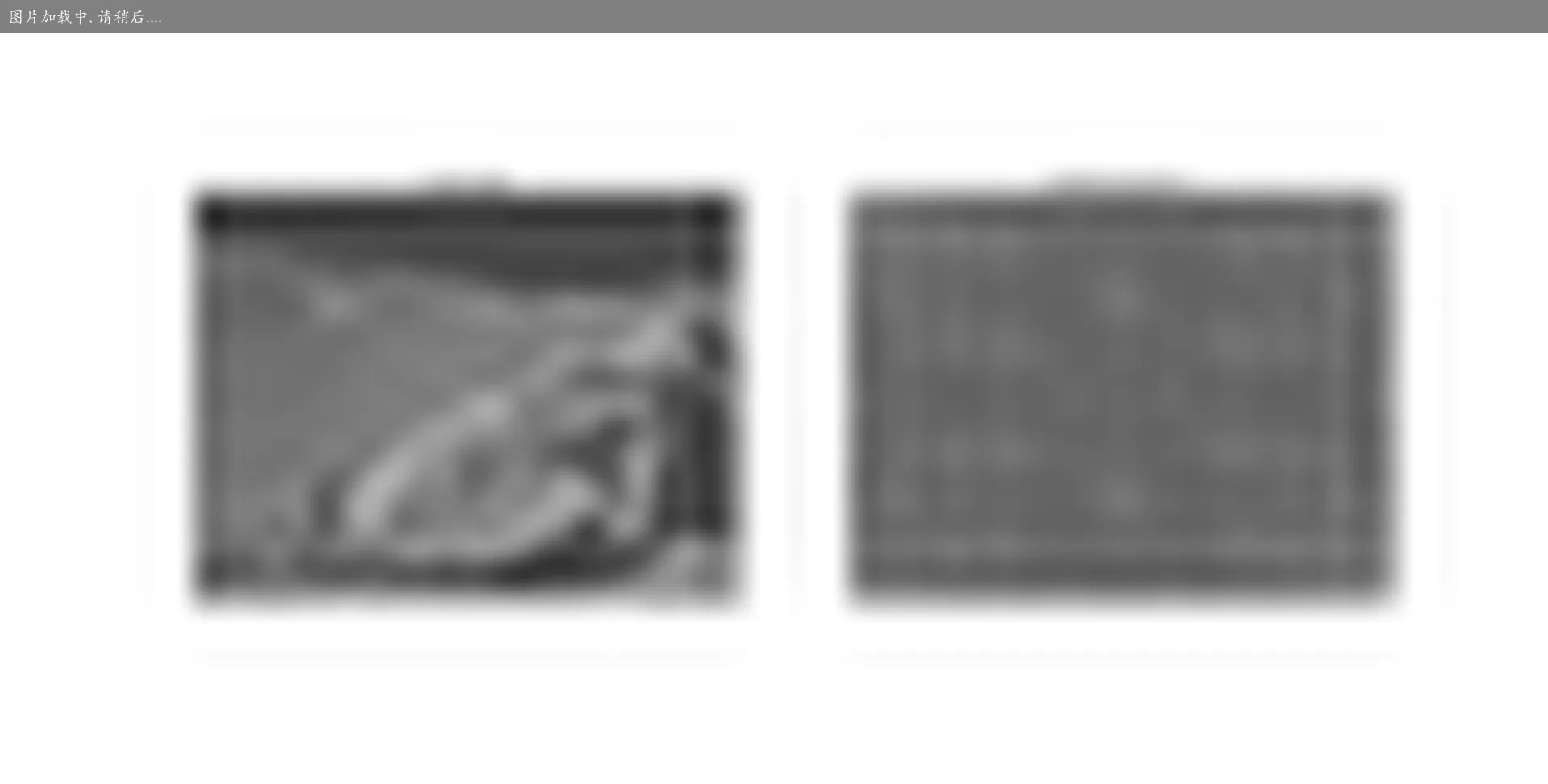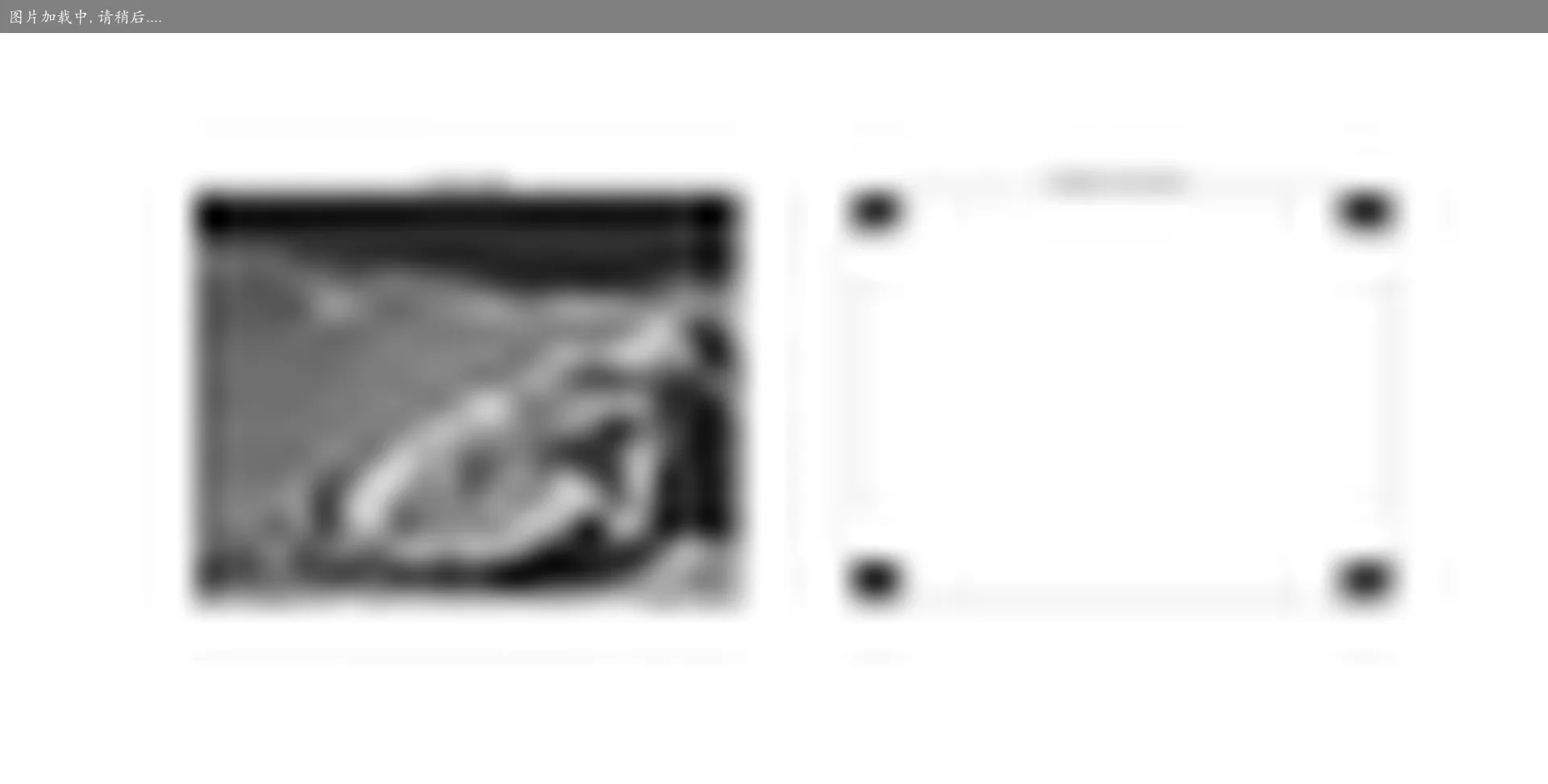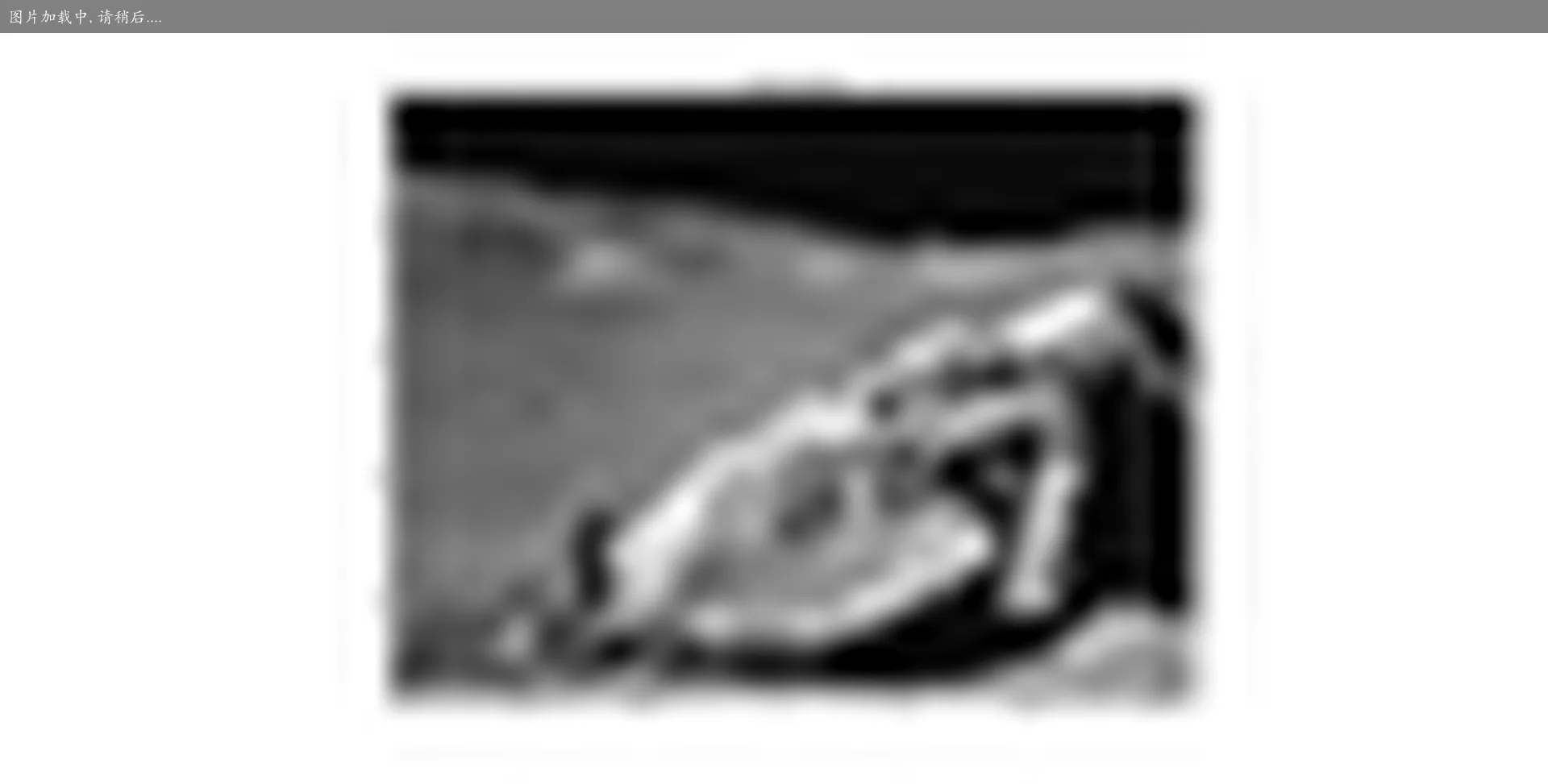## 参考

If you have any questions or feedback, please reach out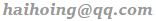.